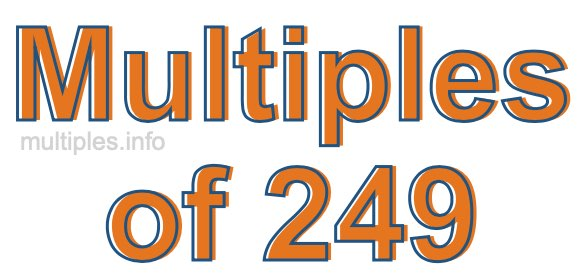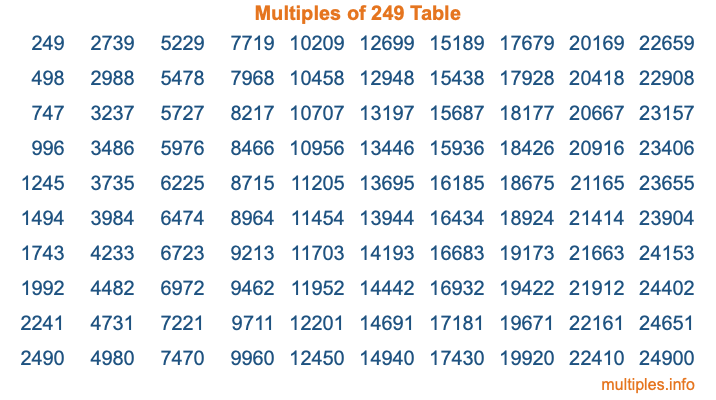Multiples of 249Welcome to the Multiples of 249 page. Here we will first teach you everything you will ever need to know about the multiples of 249, and then give you a study guide summary of everything we taught you to make sure you remember it all. Use this page to look up facts and learn information about the multiples of 249. This page will make you a multiples of two hundred forty-nine expert!

Definition of Multiples of 249
Multiples of 249 are all the numbers that when divided by 249 equal an integer. Each of the multiples of 249 are called a multiple. A multiple of 249 is created by multiplying 249 by an integer.

Therefore, to create a list of multiples of 249, you start with 1 multiplied by 249, then 2 multiplied by 249, then 3 multiplied by 249, and so on for as long as you want. Thus, the list of the first five multiples of 249 is 249, 498, 747, 996, and 1245. To see a larger list of multiples of 249, see the printable image of Multiples of 249 further down on this page. We also have a category where you can choose any nth multiple of 249.

Multiples of 249 Checker
The Multiples of 249 Checker below checks to see if any number of your choice is a multiple of 249. In other words, it checks to see if there is any number (integer) that when multiplied by 249 will equal your number. To do that, we divide your number by 249. If the the quotient is an integer, then your number is a multiple of 249.

Is  a multiple of 249?

Least Common Multiple of 249 and ...
A Least Common Multiple (LCM) is the lowest multiple that two or more numbers have in common. This is also called the smallest common multiple or lowest common multiple and is useful to know when you are adding our subtracting fractions. Enter one or more numbers below (249 is already entered) to find the LCM.

Check out our LCM Calculator if you need more details about the Least Common Multiple or if you need the LCM for different numbers for adding and subtraction fractions.

nth Multiple of 249
As we stated above, 249 is the first multiple of 249, 498 is the second multiple of 249, 747 is the third multiple of 249, and so on. Enter a number below to find the nth multiple of 249.

th multiple of 249

Multiples of 249 vs Factors of 249
249 is a multiple of 249 and a factor of 249, but that is where the similarities end. All postive multiples of 249 are 249 or greater than 249. All positive factors of 249 are 249 or less than 249.

Below is the beginning list of multiples of 249 and the factors of 249 so you can compare:

Multiples of 249: 249, 498, 747, 996, 1245, etc.

Factors of 249: 1, 3, 83, 249

As you can see, the multiples of 249 are all the numbers that you can divide by 249 to get a whole number. The factors of 249, on the other hand, are all the whole numbers that you can multiply by another whole number to get 249.

It's also interesting to note that if a number (x) is a factor of 249, then 249 will also be a multiple of that number (x).

Multiples of 249 vs Divisors of 249
The divisors of 249 are all the integers that 249 can be divided by evenly. Below is a list of the divisors of 249.

Divisors of 249: 1, 3, 83, 249

The interesting thing to note here is that if you take any multiple of 249 and divide it by a divisor of 249, you will see that the quotient is an integer.

Multiples of 249 Table
Below is an image of the first 100 multiples of 249 in a table. The table is in chronological order, column by column. The first column has the first ten multiples of 249, the second column has the next ten multiples of 249, and so on.The Multiples of 249 Table is also referred to as the 249 Times Table or Times Table of 249. You are welcome to print out our table for your studies.

Negative Multiples of 249
Although not often discussed or needed in math, it is worth mentioning that you can make a list of negative multiples of 249 by multiplying 249 by -1, then by -2, then by -3, and so on, to get the following list of negative multiples of 249:

-249, -498, -747, -996, -1245, etc.

Multiples of 249 Summary
Below is a summary of important Multiples of 249 facts that we have discussed on this page. To retain the knowledge on this page, we recommend that you read through the summary and explain to yourself or a study partner why they hold true.

There are an infinite number of multiples of 249.

A multiple of 249 divided by 249 will equal a whole number.

249 divided by a factor of 249 equals a divisor of 249.

The nth multiple of 249 is n times 249.

The largest factor of 249 is equal to the first positive multiple of 249.

249 is a multiple of every factor of 249.

249 is a multiple of 249.

A multiple of 249 divided by a divisor of 249 equals an integer.

249 divided by a divisor of 249 equals a factor of 249.

Any integer times 249 will equal a multiple of 249.

Multiples of a Number
Here you can get the multiples of another number, all with the same attention to detail as we did for multiples of 249 on this page.

Multiples of
Multiples of 250
Did you find our page about multiples of two hundred forty-nine educational? Do you want more knowledge? Check out the multiples of the next number on our list!